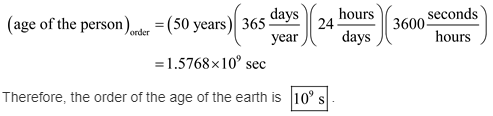# Give an order-of-magnitude estimate for the time in seconds of the following:

Give an order-of-magnitude estimate for the time in seconds of the following: (a) a year; (b) a baseball game; ( c) a heartbeat; (d) the age of the Earth; (e) the age of a person.

The power of 10 of the number which describes that quantity is called the order of magnitude.

(a)
Calculate the order of magnitude of a year as follows:
There are 60 seconds for a minute. 60 minutes for an hour. 24 hours for a day and 365 days for a year.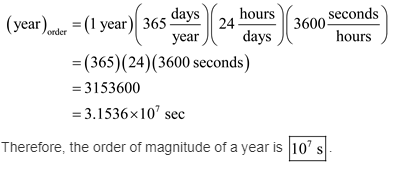(b)
Calculate the order of magnitude of a baseball as follows:
The baseball game is played for few hours that are 2 hours with 54 minutes time limit.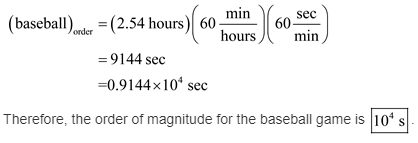( c)
Calculate the order of magnitude of a heartbeat as follows:
The heart will have 72 beats per minute.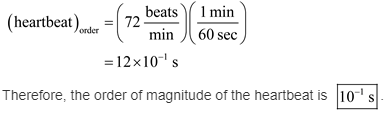(d)
Calculate the order of magnitude of the age of earth as follows: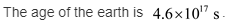On rounding off the age, the order is as follows: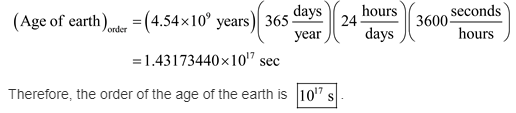(e)
Let a person can be thought of living 50 years then the order of the magnitude of time for the age of the person is,